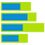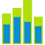# Layered chartLayered bar chartLayered column chart

A layered bar (column) chart compares two measures across one or two dimensions. The measures are shown as overlapping bars or columns (one behind the other), and they should share the same scale and unit of measurement.

If you use two dimensions for the chart, the measures are grouped by these dimensions according to their order.

For example, if you have the Country and Store Type dimensions defined in this order, the chart shows the bars or columns grouped by country, and, for each country, the bars or columns are grouped by store type.

If Store Type is the first dimension, the chart shows the bars or columns grouped by store type, and, for each store type, the bars or columns are grouped by country. However, in that case, the chart can be read easily if the number of countries is low (up to five countries).

## When to use

The layered bar (column) chart can be used to compare the following aspects:

• Gross sales and planned gross sales over time and across the store type
• The total number of motor vehicles registered compared to the number of cars registered by county (or province)

If you have two dimensions, a good rule is to set the dimension with a larger number of elements as the first dimension.

## Data requirements

To build this chart, define the data fields as follows:

• Dimensions – One or two dimensions. The order of the dimensions matters. The first dimension represents the main grouping option.
• Measures – Two measures. The order of the measures matters. The columns that represent the first measure are behind the columns that represent the second measure.
• Tooltip – (Optional) One or more measures
• Trellis – (Optional) One dimension

## Use case

View the following use cases for the layered chart, based on the chart orientation.

## References

For details on how to customize your visualization, see Visualization settings.

For a whole list of visualizations, see the following topics: